# An Extensible Proof Text Editor

Department of Computing Science
Chalmers University of Technology
S-412 96  Göteborg, Sweden

## Abstract

The paper presents an extension of the proof editor Alfa with natural-language input and output. The basis of the new functionality is an automatic translation to syntactic structures that are closer to natural language than the type-theoretical syntax of Alfa. These syntactic structures are mapped into texts in languages such as English, French, and Swedish. In this way, every theory, definition, proposition, and proof in Alfa can be translated into a text in any of these languages. The translation is defined for incomplete proof objects as well, so that a text with ``holes'' (i.e. metavariables) in it can be viewed simultaneously with a formal proof constructed. The mappings into natural language also work in the parsing direction, so that input can be given to the proof editor in a natural language.

The natural-language interface is implemented using the Grammatical Framework GF, so that it is possible to change and extend the interface without recompiling the proof editor. Such extensions can be made on two dimensions: by adding new target languages, and by adding theory-specific grammatical annotations to make texts more idiomatic.

## Introduction

Computer algebra systems, such as Mathematica [mathematica-homepage] and Maple [maple-homepage], are widely used by mathematicians and students who do not know the internals of these systems. Proof editors, such as Coq [coq-homepage], LEGO [LEGO-homepage], Isabelle [Isabelle-homepage], and ALF [magnusson:phd], are less widely used, and require more specialized knowledge than computer algebras. One important reason is, of course, that the structures involved in manipulating algebraic expressions are simpler and better understood than the structures of proofs, and typically much smaller. This difference is inescapable, and it may well be that formal proofs will never be as widely interesting as formal algebra. At the same time, there is one important factor of user-friendliness that can be improved: the language used for communication with the system. While computer algebras are reasonably conversant in the ``ordinary language'' of mathematics, that is, expressions that occur in ordinary mathematical texts, proof editors only read and write artificial languages that are designed by logicians and computer scientists but not used in mathematical texts.

Making proof editors conversant in the language of ordinary proofs is clearly a more difficult task than building support for algebraic expressions. There are two main reasons for this: first, ordinary algebraic symbolism is quite formal already, and reflects the underlying mathematical structures more closely than proof texts in books reflect the structure of proofs. Second, the realm of proofs is much wider than algebraic expressions, which is already shown by the fact that proofs can contain arbitrary algebraic expressions as parts and that they also contain many other things.

We are far from a situation in which it is possible to take an arbitrary mathematical text (even a self-contained one) and feed it into a proof editor so that the machine can check whether the proof is correct, or even return a list of open problems if the proof contains leaps too long for the machine to follow. What is within reach, however, is a restricted language at the same time intelligible to non-specialist users, formally defined, and implemented on a computer. With such a language, it is not guaranteed that the machine understands all input that the user finds meaningful, but the machine will always be able to produce output meaningful for the user.

The idea of a natural-language-like formal language of proofs was presented by de Bruijn under the title of Mathematical Vernacular [deBruijn:MV]. Implementations of such languages have been made in connection with at least Coq [coscoy:textproofs], Mizar [Mizar-homepage], and Isabelle [Isabelle-homepage]. Among these implementations, it is Coq that comes closest to the idea of having a language of proofs, in the same sense as type theory: a language in which proofs can be written, so that parts of the proof text correspond to parts of the formal proof. The other languages reflect the proof process rather than the proof object: they explain what commands the user has given to the machine, or what steps the machine has made automatically, when constructing the proof. While sometimes possibly more useful and informative than a text reflecting the proof object (because it communicates the heuristics of finding the proof), a description of the proof process is more system-dependent and less similar to ordinary proof texts than a description of the proof object.

Like the ``text extraction'' functionality of Coq [coscoy:textproofs], the present work aims to build a language of proofs whose structures are similar to the structures of proof objects. The scope of the present work is wider in certain respects:

• We do not only consider proofs but propositions and definitions as well.
• Our language can be used not only for output but for input as well ( An extension of the Coq interface [coscoy:thesis], however, has a reversible translation of proofs to texts.).
• Our language can be extended by the user in the same way as proof editors are extended by user-defined theories.
At the same time, the present work is more modest in one respect:
• We do not study automatic optimizations of the text.
The user of our interface always gets a proof text that directly reflects the formal proof, and thus has to do some extra work on the proof (and possibly on language extensions) to make the proof texts short. The Coq interface, in contrast, automatically performs certain abbreviating optimizations on the proof [coscoy:explanation]. However, the optimization feature is orthogonal to the novel features of our system, and one may well consider combining the two into something yet more powerful.

The focus of this paper is on the architecture and functionalities of a natural language interface to a proof editor. Little will be said about the linguistic questions of mathematical texts; some of the linguistic background work can be found in [ranta:torino,ranta:paris].

## Proof Editors, Type Theory and Functional Programming

Alfa [hallgren:alfa-homepage] is a graphical, syntax-directed editor for the proof system Agda. Agda [agda-homepage] is an implementation of structured type theory (STT) [coquand:stt-lfm99], which is based on Martin-Löf's type theory [martin-lof:padova]. The system is implemented completely in Haskell, using the graphical user interface toolkit Fudgets [carlsson98:fudgets_thesis].

Like its predecessors in the ALF family of proof editors [magnusson:phd], Alfa allows the user to, interactively and incrementally, define theories (axioms and inference rules), formulate theorems and construct proofs of the theorems. All steps in the proof construction are immediately checked by the system and no erroneous proofs can be constructed.

Alternatively, since Martin-Löf's type theory is a typed lambda calculus, one can view Alfa as a syntax-directed editor for a small purely functional programming language with a powerful type system.

1introexample gives an idea of what the system looks like.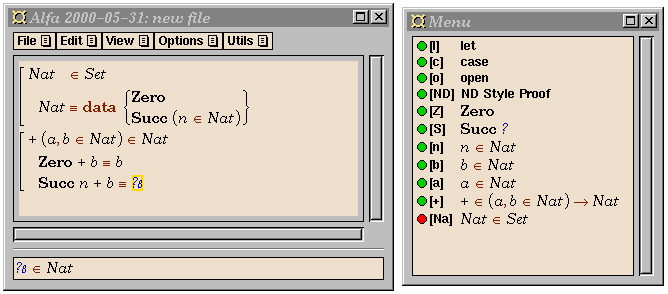introexample A window dump of Alfa.
The user has defined the natural numbers and is working on the definition of addition. Question marks are metavariables, also called place holders, and allows the user to make a definition by starting from a skeleton and gradually refine it into a complete definition in a top down fashion. When a metavariable is selected, its type is displayed at the bottom of the window, and the menu indicates which ones of the identifiers in scope may be used to construct an expression of the required type.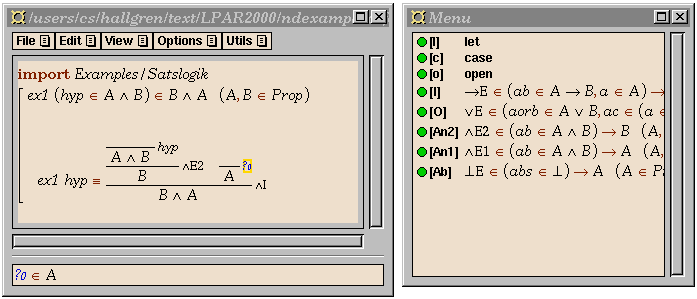ndexample A natural deduction proof in progress in Alfa.

In virtue of being based on Martin-Löf type theory, STT can draw on the Curry-Howard isomorphism and serve as a unified language for propositions and proofs, specifications and programs. This allows Alfa to be used many ways:

• As a tool for pure logic. Alfa has in fact been used in undergraduate courses, allowing the students to practice doing natural deduction style proofs in propositional logic and predicate logic. As shown in 1ndexample, Alfa has a mode of editing where terms are displayed as natural deduction style proof trees.

• As a tool for functional programming with dependent types. The language STT is closely related (The differences are to some extent due to the fact that Cayenne was designed to be used with an ordinary text editor and a batch compiler, whereas STT is designed for use in interactive proof editors.) to the language Cayenne [augustsson:cayenne], a full-fledged functional programming language with dependent types.
• As a tool for programming logic. The power of the language makes it possible to express both specifications and programs and to construct the proofs that the programs meet their specifications.

## The Grammatical Framework

the-gf

GF (Grammatical Framework) [GF-homepage] is a formalism for defining grammars. A grammar consists of an abstract syntax and a concrete syntax. The abstract syntax is a version of Martin-Löf's type theory, consisting of type and function definitions. The concrete syntax is a mapping of the abstract syntax, conceived as a free algebra, into linguistic objects. The mapping of a functional term (= abstract syntax tree) is called its linearization, since it is the flattening of a tree structure into a linear string. To give an example, the following piece of abstract syntax defines the category CN of common nouns, and two functions for forming common nouns:

```  cat CN
fun Int : CN
fun List : CN -> CN
```
To map this abstract syntax into English, we first define the class of linguistic objects corresponding to CN:
```  param Num = sg | pl
lincat CN = {s : Num => Str}
```
The first judgement introduces the parameter of number, with the two values the singular and the plural. The second judgement states that common nouns are records consisting of one field, whose type is a table of number-string pairs. The linearization rule for Int is an example of such a record ( GF uses the double arrow => for tables, or ``finite functions'', which are representable as lists of argument-value pairs. The table type is distinguished from the ordinary function type for metatheoretical reasons, such as the derivability of a parsing algorithm. A parallel distinction is made on the level of objects of these types: ordinary functions have the λ-abstract form \x →... whereas tables have the form tbl { ...}. ):
```  lin Int = {s = tbl {{sg} => "integer" ; {pl} => "integers"}}
```
In practice, it is useful to employ the GF facility of defining morphological operations, such as the inflection of regular common nouns:
```  oper regCN : Num => Str =
\str -> tbl {{sg} => str ; {pl} => str + "s"}
```
We use this operator in an equivalent linearization rule for Int, as well as in the rule for List:
```  lin Int = {s = regCN "integer"}
lin List A = {s = tbl {n => regCN "list" ! n ++ "of" ++ A.s!pl}}
```
The common noun argument of a list expression is expressed by selecting (by the table selection operator !) the plural form of the s-field of the linearization of the argument. For instance, the functional term
List (List Int)
is linearized into the record
``` {s = tbl {
{sg} => ["list of lists of natural numbers"] ;
{pl} => ["lists of lists of natural numbers"]}}
```
showing the singular and the plural forms of the complex common noun.

The concrete-syntax part of a grammar can be varied: for instance, the judgements

```  param Num = sg | pl
param Gen = masc | fem
oper regCN : Num => Str =
\str -> tbl {{sg} => str ; {pl} => str + "s"}
oper de : Str =
pre {"de" ; "d'"/strs {"a";"e";"i";"o";"u";"y"}}
lincat CN = {s : Num => Str ; g : Gen}
lin Int = {s = regCN "entier" ; g = masc}
lin List A =
{s = tbl {n => regCN "liste" ! n ++ de ++ A.s ! pl ; g = fem}}
```
define a French variant of the grammar above. Notice that, unlike English, the French rules also define a gender for common nouns, as a supplementary field of the record. ( Also notice the elision of the preposition ``de'' in front of a vowel. An ordinary linguistic processing system might treat elision by a separate morphological analyser, but the user of a proof editor may appreciate the possibility of specifying everything in one and the same source file.)

The class of grammars definable in GF includes all context-free grammars but also more ( The most important departure from context-free grammars is the possibility to permute, reduplicate, and suppress arguments of syntactic constructions. Rules using parameters, although conceptually non-context-free, can be interpreted as sets of context-free rules.). Thus GF is applicable to a wide range of formal and natural languages. The implementation of GF includes a generic algorithm of linearization, but also of parsing, that is, translating from strings back to functional terms ( The parsing algorithm is context-free parsing with some postprocessing. Suppressed arguments give rise to metavariables, which, in general, can only be restored interactively. ).

## GF-Alfa: an Interface to Alfa

gf-in-alfa

The GF interface to Alfa consists of two kinds of GF grammars:

• Core grammars, defining the translations of framework-level expressions.

• Syntactic annotations, defining translations of user-defined concepts.
The only grammar that is hard-coded in the Alfa system is the abstract syntax common to all core grammars. It is the grammar with which the normal syntax of Alfa communicates: the natural-language interface does not directly generate English or French, but expressions in this abstract syntax. The concrete syntax parts of core grammars are read from GF source when Alfa is started. Users of Alfa may thus modify them and add their own grammars for new languages ( This is relatively easy: using the English core grammar as a model, the Swedish one was constructed in less than a day. It required ca. 400 lines of GF code, of which a considerable part is not used in the core grammar itself, but consists of macros that make it easier for Alfa users to write syntactic annotations.).

The syntactic categories of the interface are, essentially, those of the syntax of type theory used in the implementation of Alfa. The most important ones are expressions, constants (=user-defined expressions), and definitions:

```  cat Exp ; Cons ; Def
```
The category Exp covers a variety of natural-language categories: common nouns, sentences, proper names, and proof texts. Rather than splitting up Exp into all these categories, we introduce a set of corresponding parameters, and state that a given expression can be linearized into all of these forms:
```  param ExpForm = cn Num | sent | pn | text ; Num = sg | pl
lincat Exp = {s : ExpForm => Str}
```
For instance, the expression emptySet, which ``intrinsically'' is a proper name, has all of these forms, of which the pn form is the shortest:
```  lin emptySet = {s = tbl {
(cn {sg}) => ["element of the empty set"] ;
(cn {pl}) => ["elements of the empty set"] ;
{sent}    => ["the empty set is inhabited"] ;
{pn}      => ["the empty set"] ;
{text}    => ["we use the empty set"]}}
```
This rule can be obtained as the result of a systematic transformation:
```  oper mkPN : Str -> {s : ExpForm => Str} = \str -> {s = tbl {
(cn {sg}) => ["element of"] ++ str ;
(cn {pl}) => ["elements of"] ++ str ;
{sent}    => str ++ ["is inhabited"] ;
{pn}      => str ;
{text}    => ["we use"] ++ str}}
lin emptySet = mkPN ["the empty set"]
```
Such transformations can be defined for each parameter value taken as the ``intrinsic'' one for a constant. The user of GF-Alfa can, to a large extent, rely on these operations and need not write explicit tables and records. However, a custom-made annotation may give more idiomatic language:
```  lin emptySet = {s = tbl {
(cn {sg}) => ["impossible element"] ;
(cn {pl}) => ["impossible elements"] ;
{sent}    => ["we have a contradiction"] ;
{pn}      => ["the empty set"] ;
{text}    => ["we use the empty set"]}}
```

The abstract syntax of the core grammars is extended every time the user defines a new concept in Alfa. The extension is by a function whose value type is Cons. For instance, the Alfa judgement

```  List (A::Set) :: Set = ...
```
is interpreted as a GF abstract syntax rule
```  fun List : Exp -> Cons
```
GF-Alfa automatically generates a default annotation,
```  lin List A = mkPN ("List" ++ A.s ! pn)
```
which the user may then edit to something more idiomatic for each target language: for instance,
```  lin List A =
mkCN (tbl {n => regCN "list" ! n ++ "of" ++ A.s ! (cn pl)})
```

The reading given to proofs is not different from other type-theoretical objects. For instance, the conjunction introduction rule, which in Alfa reads

```  ConjI (A::Set)(B::Set)(a::A)(b::B) :: Conj A B = ...
```
can be given the GF annotation
```  lin ConjI A B a b = mkText (
a.s ! text ++ "." ++ b.s ! text ++ "." ++
"Altogether" ++ A.s ! sent ++ "and" ++ B.s ! sent)
```
The rest of natural deduction rules can be treated in a similar way, using e.g. the textual forms used in [coscoy:textproofs]. It is, of course, also possible to define ad hoc inference rules and give them idiomatic linearization rules.

On the top level, an Alfa theory is a sequence of definitions. Even theorems with their proofs are definitions of constants, which linguistically correspond to names of theorems. The linearization of a definition depends on whether the constant defined is intrinsically a proper name, common noun, etc. This intrinsic feature is by default proper name, but can be changed in a syntactic annotation. In the following section, examples are given of definitions of common nouns (``natural number'') and proper names (``the sum of a and b''). Section 8 shows a definition of a constant conceived as the name of a theorem.

## Natural Language Output

The primary and most basic function of GF in Alfa is to generate natural language text from code. Any definition or expression visible in the editor window can be selected and converted into one of the supported languages by using a menu command.

As an example, the default linearization of the (completed) definitions shown in 1introexample would be as follows: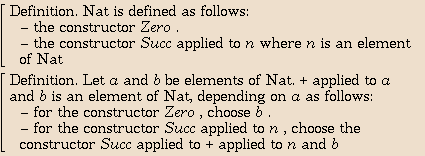By adding the following grammatical annotations,

```   Nat     = mkRegCN ["natural number"]
Zero    = mkPN "zero"
Succ n  = mkPN (["the successor of"] ++ n.s ! pn)
(+) a b = mkPN (["the sum of"] ++ a.s!pn ++ "and" ++ b.s!pn)
```

and similar grammatical annotations for Swedish and French, we obtain the following versions of the above definitions:

Definition. A natural number is defined by the following constructors:
- zero
- the successor of n where n is a natural number.
Definition. Let a and b be natural numbers. Then the sum of a and b is a natural number, defined depending on a as follows:
- for zero, choose b
- for the successor of n, choose the successor of the sum of n and b.

Définition. Les entiers naturels sont définis par les constructeurs suivants :
- zéro
- le successeur de n ou n est un entier naturel.
Définition. Soient a et b des entiers naturels. Alors la somme de a et de b est un entier naturel, qu'on définit dépendant de a de la manière suivante :
- pour zéro, choisissons b
- pour le successeur de n, choisissons le successeur de la somme de n et de b.

Definition. Ett naturligt tal definieras av följande konstruerare:
- noll
- efterföljaren till n där n är ett naturligt tal.
Definition. Låt a och b vara naturliga tal. Summan av a och b är ett naturligt tal, som definieras beroende på a enligt följande:
- för noll, välj b
- för efterföljaren till n, välj efterföljaren till summan av n och b.

It is possible to switch between the usual syntax, different language views and multilingual views by simple menu commands.

## Symbolic parts of natural-language expressions

Using natural language in every detail is not always desirable. A more suitable expression for addition, for instance, would often be

```   (+') a b = mkPN (a.s ! pn ++ "+" ++ b.s ! pn)
```
A problem with +' is, however, that it generates bad style if one or both of its arguments are expressed in natural language:
the successor of zero + 2.
The proper rule is that all parts of a symbolic expression must themselves be symbolic. This can be controlled in GF by introducing a parameter of formality and making pn dependent on it:
```   param Formality = symbolic | verbal
param ExpForm = ... | pn Formality | ...
```
The definition of mkPN must be changed so that it takes two strings as arguments, one symbolic and one verbal. We can then rephrase the annotations:
```   Zero    = mkPN "zero" "0"
(+) a b = mkPN
(["the sum of"] ++ a.s!(pn verbal) ++ "and" ++ b.s!(pn verbal))
(a.s!(pn symbolic) ++ "+" ++ b.s!(pn symbolic))
```
A separate symbolic version of + now becomes unnecessary. In a text, those parts that are to be expressed symbolically, are enclosed as arguments of an operator
```   MkSymbolic A a = mkPN (a.s!(pn symbolic)) (a.s!(pn symbolic))
```
Semantically, this operation is identity: its definition in Alfa is
```   MkSymbolic (A::Type)(a::A) = a
```
This is a typical example of an identity mapping that can be used for controlling the style of the output text.

## Natural Language Input

In addition to obtaining natural language output, you can also use the parsers automatically generated by GF to enter expressions in natural language. This way, you can make definitions without seeing any programming language syntax at all. As a simple example, suppose you want to add a definition of one as the successor of zero. By using the command to add a new definition, you get a skeleton: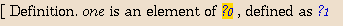The first hole to fill in is the type of one. You can use the commands ``Give in English'', ``Give in French'', ``Give in Swedish'':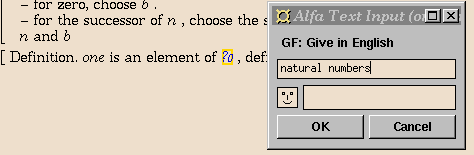The last step is to enter the body of the definition,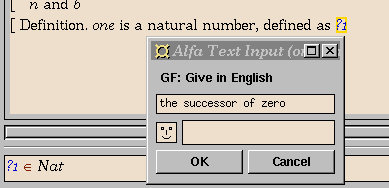and the final result is: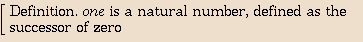The parser understands only the fragment of natural languages we have defined, but can actually correct minor grammatical errors in the input. A completion mechanism helps in finding accepted words. The smiley in the input window gives feedback from the parser.

Since GF covers arbitrary context-free grammars (and more), it is possible for the concrete syntax to be ambiguous. When an ambiguous string is entered, Alfa asks the user to choose between the resulting alternative terms.

Ambiguous structures belong intimately to natural language, including the informal language of mathematics. Banning them from the proof editor interface would thus be a drastic limitation. Syntactic ambiguity is not so disastrous as one might think: careful writers use potentially ambiguous expressions only in contexts in which they can be disambiguated. The disambiguating factor is often type checking. For instance, the English sentence

for all numbers x, x is even or x is odd
has two possible syntactic analyses, corresponding to the formulas
(x N)(Ev(x) Od(x)),

(x N)Ev(x) Od(x).

Only the first reading is actually relevant, because the second reading has an unbound variable x. In this case, the GF-Alfa interface, which filters parses through type checker, would thus not even prompt the user to choose an alternative.

Since the annotation language of GF permits the user to introduce ambiguous structures, the parsing facility plays an important role even in natural language output: the question whether a text generated from a proof is ambiguous can be answered by parsing the text. Even a user who does not care about the natural language input facility of GF-Alfa may want to use the GF parser to find ambiguities in natural language output.

## An Example: Insertion Sort

sorting-example

As a small, but non-trivial, example where GF and many features of Alfa are used together, we show some fragments from a correctness proof of a sorting algorithm.

We have defined insertion sort for lists of natural numbers in the typical functional programming style: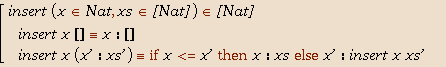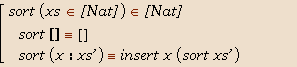The English translation of the definition of sort is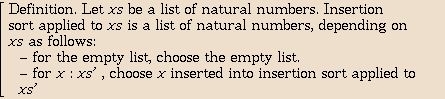As a specification of the sorting problem, we use the following: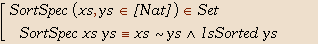We have chosen ``ys is a sorted version of xs'' as the English translation of SortSpec xs ys. The body of SortSpec translates to ``ys is a permutation of xs and ys is sorted''.

After proving some properties about permutations and the insert function, we can fairly easily construct the correctness proof for sort by induction on the list to be sorted. The proof is shown in natural deduction style in 1sortiscorrectnd.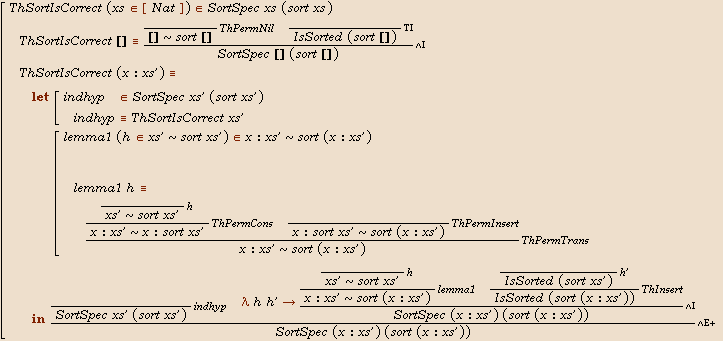sortiscorrectnd The correctness proof for insertion sort. See section sorting-example.
The specification SortSpec xs ys is defined to mean that ys is a permutation of xs, denoted xs ~ys, and ys is sorted, denoted IsSorted ys.

The same proof can also be viewed in English. The beginning of it is: (We omit the rest of the proof for the time being. Some fine tuning is needed to make the text look really nice.)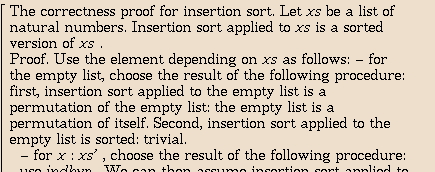Using the above proof, we can easily prove the proposition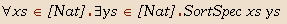The English translation of the proof is: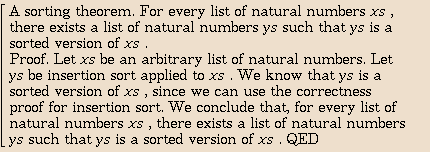## Conclusion

While Alfa dates back to 1995 and GF to 1998, the work on GF-Alfa only started at the end of 1999. It has been encouraging that the overall concept of integrating GF and Alfa works. Moreover, there is nothing particular to Alfa that makes this type of interface work; an earlier interface with the same architecture (i.e. core grammar + syntactic annotations) was built for the completely different formalism of extended regular expressions [ranta98:regexp]. Similar lessons can be learnt from both systems:

• Formal structures can be mapped to natural-language structures so that arbitrarily complex expressions always give grammatically correct results. Thus it is possible to translate from formal to natural languages.

• Complex expressions are harder to understand in natural than in formal languages. Thus it is important to structure the code and break it into small units (such as lemmas), in order for the resulting text to be readable.

• It is useful to define equivalent variants of formal objects and equip them with different linearization rules. In this way stylistic variation can be included in the text. Linearization rules can also implement different degrees of information hiding.

• Natural-language input is only useful for small expressions, since entering a long expression runs the risk of falling outside the grammar.

• The interactive construction of a formal object is helped by a simultaneous view of the object as informal text.

• Ambiguities need not be forbidden in natural language, since they can be handled by interaction. Moreover, syntactic ambiguities are often automatically resolved by type checking.
The technique of improving the style of generated texts by syntactic annotations is interactive rather than automatic. Thus it fits well in the concept of interactive proof editors. This does not exclude the possibility of automatic text optimizations, e.g. factorizing parts of texts into shared parts (cf. [coscoy:explanation]). The preferable place of such operations is on the level of abstract syntax, from where they are propagated to all target languages. Language-specific optimizations would certainly enable more elegant texts to be produced, but they would at the same time reduce the extensibility of the system.

## References

coq-homepage Coq Homepage.
http://pauillac.inria.fr/coq/, 1999.

LEGO-homepage The LEGO Proof Assistant.
http://www.dcs.ed.ac.uk/home/lego/, 1999.

Mizar-homepage The Mizar Homepage.
http://mizar.org/, 1999.

Isabelle-homepage Isabelle Homepage.
http://www.cl.cam.ac.uk/Research/HVG/Isabelle/, 2000.

augustsson:cayenne Lennart Augustsson.
Cayenne --- a language with dependent types.
In Proc. of the International Conference on Functional Programming (ICFP'98). ACM Press, September 1998.

carlsson98:fudgets_thesis M. Carlsson and T. Hallgren.
Fudgets --- Purely Functional Processes with applications to Graphical User Interfaces.
PhD thesis, Department of Computing Science, Chalmers University of Technology, S-412 96 Göteborg, Sweden, March 1998.

agda-homepage C. Coquand.
AGDA Homepage.
http://www.cs.chalmers.se/~catarina/agda/, 1998.

coquand:stt-lfm99 C. Coquand and T. Coquand.
Structured type theory.
In Workshop on Logical Frameworkds and Meta-languages, Paris, France, Sep 1999.

coscoy:explanation Y. Coscoy.
A natural language explanation of formal proofs.
In C. Retoré, editor, Logical Aspects of Computational Linguistics, number 1328 in Lecture Notes in Artificial Intelligence, pages 149--167, Heidelberg, 1997. Springer.

coscoy:thesis Y. Coscoy.
Explication textuelle de preuves pour le calcul des constructions inductives.
PhD thesis, Université de Nice-Sophia-Antipolis, 2000.

coscoy:textproofs Y. Coscoy, G. Kahn, and L. Théry.
Extracting text from proof.
In M. Dezani and G. Plotkin, editors, Proceedings of the International Conference on Typed Lambda Calculus and Applications (TLCA), Edinburgh, number 902 in Lecture Notes in Computer Science. Springer-Verlag, 1996.

deBruijn:MV N. G. de Bruijn.
Mathematical Vernacular: a Language for Mathematics with Typed Sets.
In R. Nederpelt, editor, Selected Papers on Automath, pages 865--935. North-Holland Publishing Company, 1994.

hallgren:alfa-homepage T. Hallgren.
http://www.cs.chalmers.se/~hallgren/Alfa/, 1996-2000.

maple-homepage Waterloo Maple Inc.
Maple Homepage.
http://www.maplesof.com/, 2000.

magnusson:phd L. Magnusson.
The Implementation of ALF - a Proof Editor based on Martin-Löf's Monomorphic Type Theory with Explicit Substitution.
PhD thesis, Department of Computing Science, Chalmers University of Technology and University of Göteborg, 1994.

Intuitionistic Type Theory.
Bibliopolis, Napoli, 1984.

ranta:torino A. Ranta.
Context-relative syntactic categories and the formalization of mathematical text.
In S. Berardi and M. Coppo, editors, Types For Proofs and Programs, number 1158 in Lecture Notes in Computer Science, pages 231--248. Springer-Verlag, 1996.

ranta:paris A. Ranta.
Structures grammaticales dans le français mathématique.
Mathématiques, informatique et Sciences Humaines, (138, 139):5--56, 5--36, 1997.

ranta98:regexp A. Ranta.
A multilingual natural-language interface to regular expressions.
In L. Karttunen and K. Oflazer, editors, Proceedings of the International Workshop on Finite State Methods in Natural Language Processing, pages 79--90, Ankara, 1998. Bilkent University.

GF-homepage A. Ranta.
Grammatical Framework Homepage.
http://www.cs.chalmers.se/~aarne/GF/index.html, 2000.

mathematica-homepage Inc. Wolfram Research.
Mathematica Homepage.
http://www.wolfram.com/products/mathematica/, 2000.